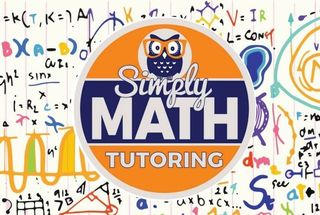\$749.00 - \$749.00

# 6th Grade – 4 week Math BootCamp• Ages:

Details of 4 week Math BootCamp:

Whole numbers

→    Place values in whole numbers

→    Word names for numbers

→    Roman numerals

→    Add and subtract whole numbers

Multiplication

→    Multiply whole numbers

→    Multiply whole numbers: word problems

→    Multiply numbers ending in zeroes

→    Multiply numbers ending in zeroes: word problems

Division

→    Divisibility rules

→    Division patterns with zeroes

→    Divide numbers ending in zeroes: word problems

Integers

→    Understanding integers

→    Integers on number lines

→    Graph integers on horizontal and vertical number lines

→    Compare and order integers

Operations with integers

→    Subtract integers using counter

→    Subtract integers

→    Add and subtract integers: find the sign

Expressions and properties

→    Write variable expressions

→    Evaluate variable expressions

→    Properties of addition and multiplication

→    Multiply using the distributive property

→    Write equivalent expressions using properties

→    Properties of multiplication

One-variable equations

→    Write variable equations: word problems

→    Solve equations using properties

Constructions

→    Construct the midpoint or perpendicular bisector of a segment

→    Construct an angle bisector

→    Construct a perpendicular line